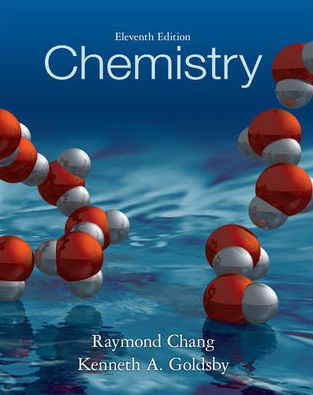×
Get Full Access to Chemistry - 11 Edition - Chapter 7 - Problem 73p
Get Full Access to Chemistry - 11 Edition - Chapter 7 - Problem 73p

×

# Solved: Explain the meaning of diamagnetic andISBN: 9780073402680 118

## Solution for problem 73P Chapter 7

Chemistry | 11th Edition

• Textbook Solutions
• 2901 Step-by-step solutions solved by professors and subject experts
• Get 24/7 help from StudySoup virtual teaching assistantsChemistry | 11th Edition

4 5 1 259 Reviews
25
5
Problem 73P

Explain the meaning of diamagnetic and paramagnetic. Give an example of an element that is diamagnetic and one that is paramagnetic. What does it mean when we say that electrons are paired?

Step-by-Step Solution:

Step 1 of 4

Here we have to explain the meaning of diamagnetic and paramagnetic along with this we have to give an example of an element that is diamagnetic and one that is paramagnetic. What does it mean when we say that electrons are paired?

Step 2 of 4

Step 3 of 4

##### ISBN: 9780073402680

This textbook survival guide was created for the textbook: Chemistry, edition: 11. The full step-by-step solution to problem: 73P from chapter: 7 was answered by , our top Chemistry solution expert on 11/08/17, 03:59AM. This full solution covers the following key subjects: diamagnetic, paramagnetic, give, Example, explain. This expansive textbook survival guide covers 25 chapters, and 3591 solutions. The answer to “?Explain the meaning of diamagnetic and paramagnetic. Give an example of an element that is diamagnetic and one that is paramagnetic. What does it mean when we say that electrons are paired?” is broken down into a number of easy to follow steps, and 32 words. Chemistry was written by and is associated to the ISBN: 9780073402680. Since the solution to 73P from 7 chapter was answered, more than 304 students have viewed the full step-by-step answer.

## Discover and learn what students are asking

Calculus: Early Transcendental Functions : Inverse Trigonometric Functions: Integration
?In Exercises 1-20, find the indefinite integral. $$\int \frac{t}{t^{4}+25} d t$$

Unlock Textbook Solution

Enter your email below to unlock your verified solution to:

Solved: Explain the meaning of diamagnetic and